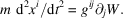International
Tables for
Crystallography
Volume D
Physical properties of crystals
Edited by A. Authier

International Tables for Crystallography (2006). Vol. D, ch. 1.1, p. 9

## Section 1.1.3.6. Change of variance of the components of a tensor

A. Authiera*

aInstitut de Minéralogie et de la Physique des Milieux Condensés, Bâtiment 7, 140 rue de Lourmel, 75015 Paris, France
Correspondence e-mail: aauthier@wanadoo.fr

| top | pdf |

#### 1.1.3.6.1. Tensor nature of the metric tensor

| top | pdf |

Equation (1.1.2.17)describing the behaviour of the quantities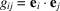under a change of basis shows that they are the components of a tensor of rank 2, the metric tensor. In the same way, equation (1.1.2.19)shows that the's transform under a change of basis like the product of two contravariant coordinates. The coefficientsandare the components of a unique tensor, in one case doubly contravariant, in the other case doubly covariant. In a general way, the Euclidean tensors (constructed in a space where one has defined the scalar product) are geometrical entities that can have covariant, contravariant or mixed components.

#### 1.1.3.6.2. How to change the variance of the components of a tensor

| top | pdf |

Let us take a tensor product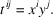We know that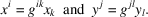It follows that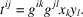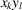is a tensor product of two vectors expressed in the dual space: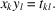One can thus pass from the doubly covariant form to the doubly contravariant form of the tensor by means of the relation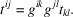This result is general: to change the variance of a tensor (in practice, to raise or lower an index), it is necessary to make the contracted product of this tensor usingor, according to the case. For instance,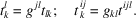Remark.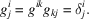This is a property of the metric tensor.

#### 1.1.3.6.3. Examples of the use in physics of different representations of the same quantity

| top | pdf |

Let us consider, for example, the force, F, which is a tensor quantity (tensor of rank 1). One can define it:

 (i) by the fundamental law of dynamics: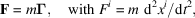where m is the mass and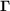is the acceleration. The force appears here in a contravariant form. (ii) as the derivative of the energy, W: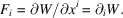The force appears here in covariant form. In effect, we shall see in Section 1.1.3.8.1that to form a derivative with respect to a variable contravariant augments the covariance by unity. The general expression of the law of dynamics is therefore written with the energy as follows: